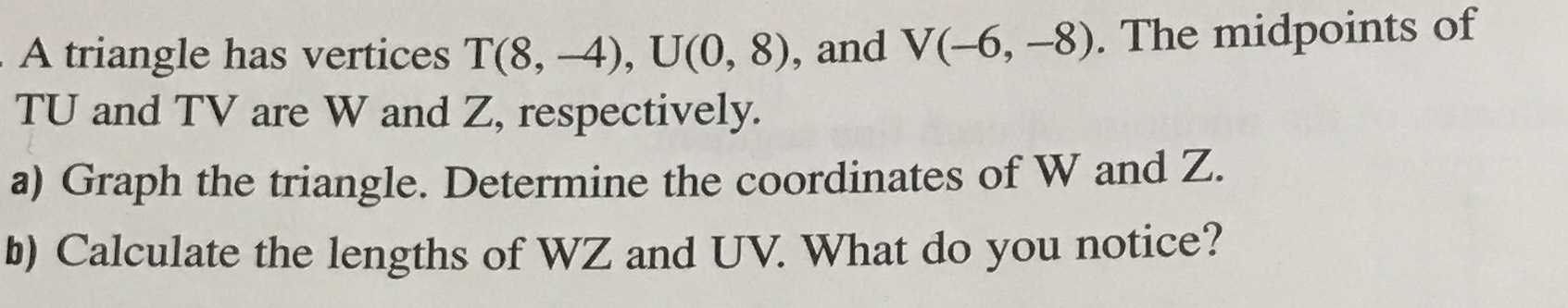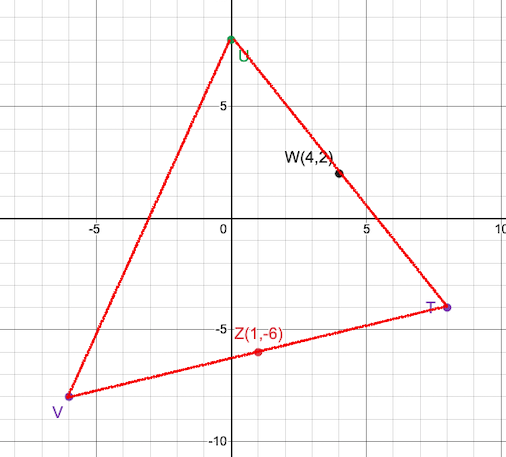### ¿Todavía tienes preguntas de matemáticas?

Pregunte a nuestros tutores expertos
Trigonometry
PreguntaA triangle has vertices $$T ( 8 , - 4 ) , U ( 0,8 ) ,$$ and $$V ( - 6 , - 8 )$$ . The midpoints of

$$TU$$ and $$TV$$ are $$W$$ and $$Z$$ , respectively. a) Graph the triangle. Determine the coordinates of $$W$$ and $$Z$$ . b) Calculate the lengths of $$WZ$$ and $$UV$$ . What do you notice?

a)b)

WZ=√73

UV=2√73

the length of WZ is half of the length of UV

Solución
View full explanation on CameraMath App.